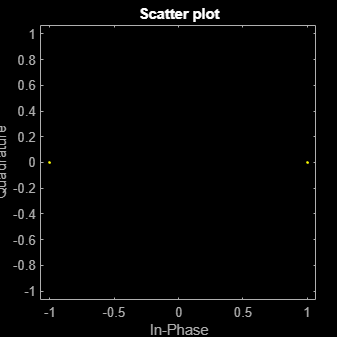wlanConstellationMap

Constellation mapping

Description

example

y = wlanConstellationMap(bits,numBPSCS) maps input sequence bits using numBPSCS, the number of coded bits per subcarrier per spatial stream.

example

y = wlanConstellationMap(bits,numBPSCS,phase) rotates the constellation points counterclockwise by the number of radians specified in phase.

Examples

collapse all

Perform a 4096-QAM mapping.

Create the sequence of data bits.

bits = randi([0 1],49152,1,'int8');

Perform the constellation mapping on the data bits with 4096-QAM.

numBPSCS = 12;
y = wlanConstellationMap(bits,numBPSCS);

The size of the output returned by this modulation is the size of the input sequence divided by 12.

size(y)
ans = 1×2

4096           1

Perform $\frac{\pi }{2}$-BPSK mapping on a sequence of data bits.

Create the sequence of data bits.

bits = randi([0 1],512,1);

Perform the BPSK mapping on the data bits with a rotation of $\frac{\pi }{2}$ radians.

numBPSCS = 1;
phase = pi*(0:size(bits,1)/numBPSCS-1).'/2;
y = wlanConstellationMap(bits,numBPSCS,phase);

The size of the output is equal to the size of the original sequence.

size(y)
ans = 1×2

512     1

Display the modulated signal constellation.

scatterplot(y)Perform BPSK mapping of OFDM symbols for the VHT-SIG-A field.

Create the sequence of data bits. Place the two OFDM symbols in columns.

bits = randi([0 1],48,2,'int8');

Perform constellation mapping on the data bits. Specify the size of constellation rotation phase as the number of columns in the input sequence.

numBPSCS = 1;
phase = [0 pi/2];
y = wlanConstellationMap(bits,numBPSCS,phase);

Display the modulated signal constellation.

scatterplot(y(:,1))scatterplot(y(:,2))Input Arguments

collapse all

Input sequence of bits to map into symbols, specified as a binary-valued vector, matrix, or multidimensional array.

Data Types: double | int8

Number of coded bits per subcarrier per spatial stream, specified as log2(M), where M is the modulation order. Therefore, numBPSCS must be one of these values.

• 1 for binary phase-shift keying (BPSK) modulation, as specified in section 17.3.5.8 of 

• 2 for quadrature phase-shift keying (QPSK) modulation, as specified in section 17.3.5.8 of 

• 4 for 16-point quadrature amplitude modulation (16-QAM), as specified in section 17.3.5.8 of 

• 6 for 64-QAM, as specified in section 17.3.5.8 of 

• 8 for 256-QAM, as specified in section 21.3.10.9 of 

• 10 for 1024-QAM, as specified in section 27.3.11.9 of 

• 12 for 4096-QAM, as specified in section 36.3.12.8 of 

Example: 4

Data Types: double

Constellation rotation in radians, specified as a scalar, vector, or multidimensional array. The size of phase must be compatible with the size of the bits input. This input and bits have compatible sizes if, for each corresponding dimension, the dimension sizes are either equal or one of them is 1. When one of the dimensions of bits is equal to 1 and the corresponding dimension of phase is larger than 1, then the output dimensions have the same size as the dimensions of phase.

Example: pi*(0:size(bits,1)/numBPSCS-1).'/2;

Data Types: double

Output Arguments

collapse all

Mapped symbols, returned as a complex-valued vector, matrix, or multidimensional array. This output has the same size as bits, except for the number of rows, which is equal to the number of rows of bits divided by numBPSCS.

Data Types: double
Complex Number Support: Yes

 IEEE Std 802.11™-2016 (Revision of IEEE Std 802.11-2012). “Part 11: Wireless LAN Medium Access Control (MAC) and Physical Layer (PHY) Specifications.” IEEE Standard for Information technology — Telecommunications and information exchange between systems. Local and metropolitan area networks — Specific requirements.

 IEEE P802.11ax™/D4.1. “Part 11: Wireless LAN Medium Access Control (MAC) and Physical Layer (PHY) Specifications. Amendment 1: Enhancements for High Efficiency WLAN.” Draft Standard for Information technology — Telecommunications and information exchange between systems. Local and metropolitan area networks — Specific requirements.

 IEEE® P802.11be™/D1.0. “Part 11: Wireless LAN Medium Access Control (MAC) and Physical Layer (PHY) Specifications. Amendment 8: Enhancements for Extremely High Throughput (EHT).” Draft Standard for Information technology — Telecommunications and information exchange between systems. Local and metropolitan area networks — Specific requirements.

WLAN Toolbox DocumentationGet trial now